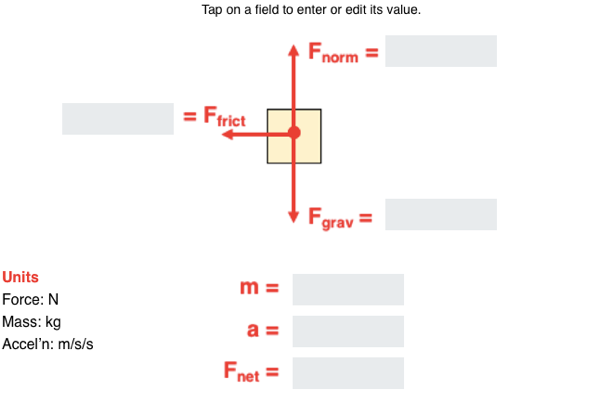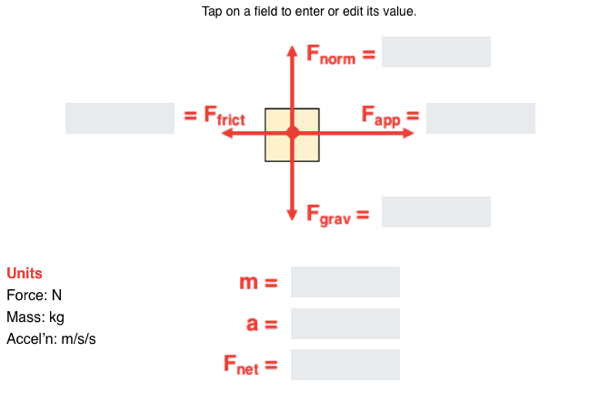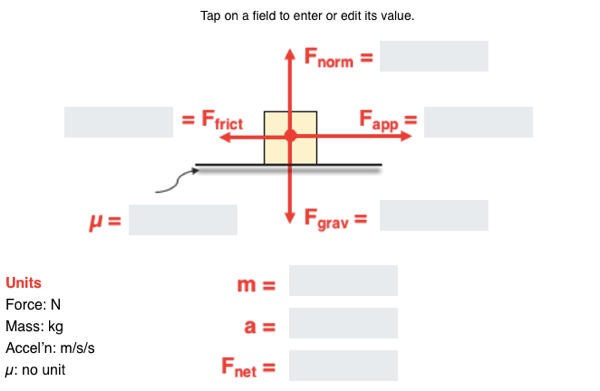## Solve It! (with Newton's Second Law) - Questions

The Solve It! (with Newton's Second Law) Concept Builder is comprised of 8 problems. Each problem differs in terms of the given quantities and the unknown quantities. The numerical values in the problem are randomly generated. There are three difficulty levels for these problems. The difficulty levels are differentiated by the complexity of the problem as follows:

• Apprentice Level: Problems 1-2; includes three forces and six answer blanks.
• Masters Level: Problems 3-5; includes four forces and seven answer blanks.
• Wizard Level: Problems 6-8; includes four forces, a coefficient of friction, and eight answer blanks.

Sample problems are shown below. The actual numerical values will vary each time the problem is displayed on the screen. Teachers are encouraged to view the problems in order to judge which level of difficulty is most appropriate for their classes. We recommend providing students two or more options. For instance, it would be profitable for a student to complete the Concept Builder at the Apprentice Level in order to gain some confidence and then to be challenged to complete the activity at the Masters Level.

The Physics Classroom grants teachers and other users the right to print these questions for private use. Users are also granted the right to copy the text and modify it for their own use. However, this document should not be uploaded to other servers for distribution to and/or display by others. The Physics Classroom website should remain the only website or server from which the document is distributed or displayed. We also provide a PDF that teachers can use under the same conditions. We have included a link to the PDF near the bottom of this page.

#### Solve It! (with Newton’s Second Law)

NOTE: Numerical values used in this Concept Builder are randomly generated and likely different than those published here.

Apprentice Difficulty Level
Question 1
Analyze this: A frictional force of 22.5 N acts upon a 3.86-kg rightward-moving box to accelerate it leftward. Complete the diagram.Question 2
Analyze this:  A frictional force acts upon a 27.2-kg rightward-moving box to accelerate it leftward at 5.42 m/s/s. Complete the diagram.Master Difficulty Level
Question 3
Analyze this: A rightward force of 83.2 N is applied to a 5.39-kg object to accelerate it to the right. The friction force between the object and the surface is 32.7 N. Complete the diagram.Question 4
Analyze this: A rightward force of 453 N is applied to a 25.3-kg object to accelerate it to the right at 13.4 m/s/s. Complete the diagram.Question 5
Analyze this: A rightward force is applied to a 6.85-kg object to accelerate it to the right at 9.21 m/s/s. The friction force between the object and the surface is 33.4 N. Complete the diagram.Wizard Difficulty Level
Question 6
Analyze this: A rightward force is applied to a 27.5-kg object to accelerate it to the right at 17.9 m/s/s. The coefficient of friction for the object and the surface is 0.353. Complete the diagram.Question 7
Analyze this: A rightward force of 332 N is applied to a 24.7-kg object to accelerate it to the right. The coefficient of friction for the object and the surface is 0.564. Complete the diagram.Question 8
Analyze this:  A rightward force of 62.9 N is applied to a 7.54-kg object to accelerate it to the right at 4.77 m/s/s. Complete the diagram.